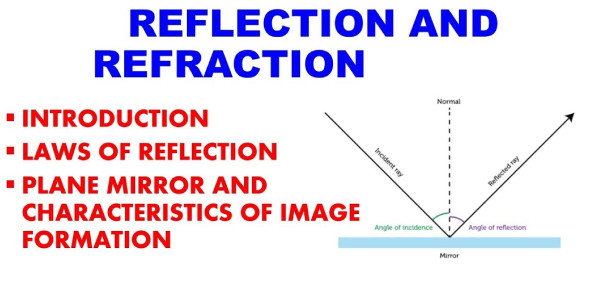# Reflection And Refraction Quiz: Physics Trivia!

10 Questions | Total Attempts: 555SettingsThis is a physics trivia about reflection and refraction. A lot of people have a hard time differentiating between these two methods. Both methods have waves, but while in one, the wave direction changes as it passes through a medium while in the other, the wavefront returns into the medium it originated. Can you comfortably notice which method is which and how they occur by taking this quiz? Check it out!

Related Topics
• 1.
A girl looks into a bathroom mirror to brush her hair. The figure shows what she sees in the mirror. On which hand is she holding the brush?
• A.

Right hand

• B.

Left hand

• 2.
What causes refraction when light travels from air into glass?
• A.

The amplitude of the light waves changes.

• B.

The colour of the light changes.

• C.

The frequency of the light waves changes.

• D.

The speed of the light changes.

• 3.
The diagram shows a ray of light entering a block of glass.Which numbered angles are the angles of incidence and of refraction?
• A.

Incidence:1; refraction: 3

• B.

Incidence:1; refraction: 4

• C.

Incidence:2; refraction: 3

• D.

Incidence:2; refraction: 4

• 4.
• A.

A

• B.

B

• C.

C

• D.

D

• 5.
Light from the Sun passes through a prism and a spectrum is produced on a screen. A thermometer placed at P shows a large temperature rise. Which type of radiation causes this?
• A.

Infra-red

• B.

Microwave

• C.

Ultra-violet

• D.

Visible light

• 6.
The diagram shows a ray of light incident on the edge of a piece of glass. The angle i is bigger than the critical angle. Which arrow correctly shows the direction of the ray after it leaves the edge of the glass?
• A.

A

• B.

B

• C.

C

• D.

D

• 7.
A man sees a stone at the bottom of a pool of water. Which path could be taken by light from the stone to the man?
• A.

A

• B.

B

• C.

C

• D.

D

• 8.
A ray of light passes from air into a block of glass with an index of refraction of 1.5. The angle of incidence is 30 degrees. Calculate the angle of refraction.
• A.

30

• B.

19.5

• C.

0.05

• D.

1

• 9.
The speed of light in air is almost 3x108 m/s. In one piece of glass, light slows down to 2x108 m/s. What is the index of refraction for the glass?
• A.

6

• B.

0.67

• C.

1.5

• D.

5

• 10.
In an optics lesson, a Physics student traces the paths of three rays of light near the boundary between medium A and air. The student uses a protractor to measure the various angles. State the critical angle of medium A
• A.

53

• B.

90

• C.

133

• D.

43